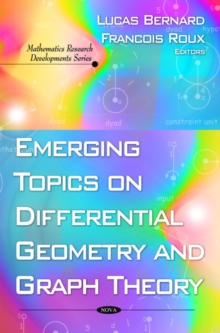Supporting your high street Find out how »
• My AccountBasket# Emerging Topics on Differential Geometry & Graph Theory Hardback

## Edited by Lucas Bernard, Francois Roux

#### Description

Differential geometry is a mathematical discipline that uses the methods of differential and integral calculus to study problems in geometry.

Graph theory is also a growing area in mathematical research.

In mathematics and computer science, graph theory is the study of mathematical structures used to model pairwise relations between objects from a certain collection.

This book presents various theories and applications in both of these mathematical fields.

Included are the concepts of dominating sets, one of the most widely studied concepts in graph theory, some current developments of graph theory in the fields of planar linkage mechanisms and geared linkage mechanisms, lie algebras and the application of CR Hamiltonian flows to the deformation theory of CR structures.

#### Information

• Format: Hardback
• Pages: 417 pages, tables & charts
• Publisher: Nova Science Publishers Inc
• Publication Date:
• Category: Differential & Riemannian geometry
• ISBN: 9781607410119

£109.99

£89.09

on all orders

###### Pick up orders

from local bookshops